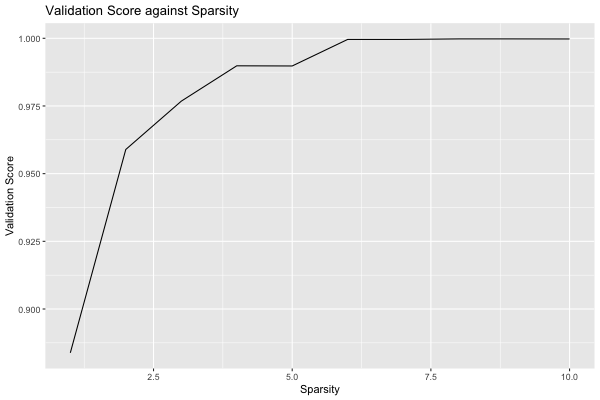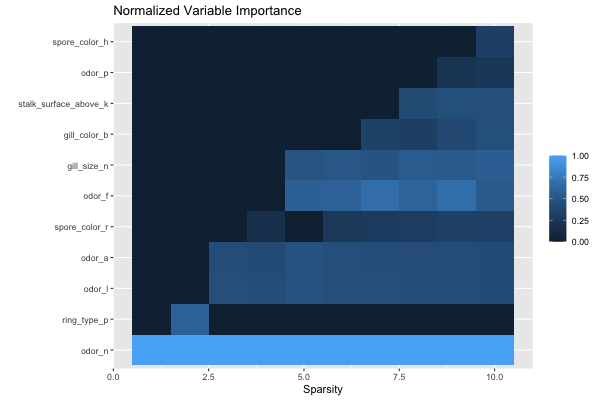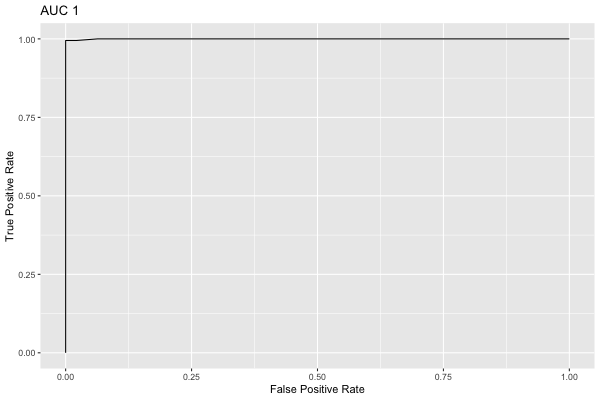# Quick Start Guide: Optimal Feature Selection for Classification

This is an R version of the corresponding OptimalFeatureSelection quick start guide.

In this example we will use Optimal Feature Selection on the Mushroom dataset, where the goal is to distinguish poisonous from edible mushrooms.

First we load in the data and split into training and test datasets:

df <- read.table(
"agaricus-lepiota.data",
sep = ",",
col.names = c("target", "cap_shape", "cap_surface", "cap_color",
"bruises", "odor", "gill_attachment", "gill_spacing",
"gill_size", "gill_color", "stalk_shape", "stalk_root",
"stalk_surface_above", "stalk_surface_below",
"stalk_color_above", "stalk_color_below", "veil_type",
"veil_color", "ring_number", "ring_type", "spore_color",
"population", "habitat"),
stringsAsFactors = T,
)

  target cap_shape cap_surface cap_color bruises odor gill_attachment
1      p         x           s         n       t    p               f
2      e         x           s         y       t    a               f
gill_spacing gill_size gill_color stalk_shape stalk_root stalk_surface_above
1            c         n          k           e          e                   s
2            c         b          k           e          c                   s
stalk_surface_below stalk_color_above stalk_color_below veil_type veil_color
1                   s                 w                 w         p          w
2                   s                 w                 w         p          w
ring_number ring_type spore_color population habitat
1           o         p           k          s       u
2           o         p           n          n       g
[ reached 'max' / getOption("max.print") -- omitted 8122 rows ]
X <- df[, 2:23]
y <- df[, 1]
split <- iai::split_data("classification", X, y, seed = 1)
train_X <- split$train$X
train_y <- split$train$y
test_X <- split$test$X
test_y <- split$test$y


### Model Fitting

We will use a grid_search to fit an optimal_feature_selection_classifier:

grid <- iai::grid_search(
iai::optimal_feature_selection_classifier(
random_seed = 1,
),
sparsity = 1:10,
)
iai::fit(grid, train_X, train_y, validation_criterion = "auc")

Julia Object of type GridSearch{OptimalFeatureSelectionClassifier, IAIBase.NullGridResult, IAIBase.Data{IAIBase.ClassificationTask, IAIBase.ClassificationTarget}}.
All Grid Results:

Row │ sparsity  train_score  valid_score  rank_valid_score
│ Int64     Float64      Float64      Int64
─────┼──────────────────────────────────────────────────────
1 │        1     0.530592     0.883816                10
2 │        2     0.666962     0.958933                 9
3 │        3     0.852881     0.97677                  8
4 │        4     0.886338     0.989837                 6
5 │        5     0.8786       0.989776                 7
6 │        6     0.918052     0.999586                 4
7 │        7     0.921551     0.999586                 5
8 │        8     0.930723     0.999783                 1
9 │        9     0.933428     0.999783                 2
10 │       10     0.933968     0.999755                 3

Best Params:
sparsity => 8

Best Model - Fitted OptimalFeatureSelectionClassifier:
Constant: -0.102149
Weights:
gill_color=b:           1.30099
gill_size=n:            2.15205
odor=a:                -3.56147
odor=f:                 2.41679
odor=l:                -3.57161
odor=n:                -3.58869
spore_color=r:          6.1136
stalk_surface_above=k:  1.56583
(Higher score indicates stronger prediction for class p)

The model selected a sparsity of 8 as the best parameter, but we observe that the validation scores are close for many of the parameters. We can use the results of the grid search to explore the tradeoff between the complexity of the regression and the quality of predictions:

plot(grid, type = "validation")We see that the quality of the model quickly increases with as features are adding, reaching AUC 0.98 with 3 features. After this, additional features increase the quality more slowly, eventually reaching AUC close to 1 with 6 features. Depending on the application, we might decide to choose a lower sparsity for the final model than the value chosen by the grid search.

We can see the relative importance of the selected features with variable_importance:

iai::variable_importance(iai::get_learner(grid))

                 Feature Importance
1                 odor=n 0.24741179
2                 odor=f 0.14800069
3            gill_size=n 0.13889144
4                 odor=l 0.10930307
5                 odor=a 0.10554711
6  stalk_surface_above=k 0.09867952
7          spore_color=r 0.07780573
8           gill_color=b 0.07436066
9              bruises=t 0.00000000
10           cap_color=b 0.00000000
11           cap_color=c 0.00000000
12           cap_color=e 0.00000000
13           cap_color=g 0.00000000
14           cap_color=n 0.00000000
15           cap_color=p 0.00000000
16           cap_color=r 0.00000000
17           cap_color=u 0.00000000
18           cap_color=w 0.00000000
19           cap_color=y 0.00000000
20           cap_shape=b 0.00000000
21           cap_shape=c 0.00000000
22           cap_shape=f 0.00000000
23           cap_shape=k 0.00000000
24           cap_shape=s 0.00000000
25           cap_shape=x 0.00000000
26         cap_surface=f 0.00000000
27         cap_surface=g 0.00000000
28         cap_surface=s 0.00000000
29         cap_surface=y 0.00000000
30     gill_attachment=f 0.00000000
[ reached 'max' / getOption("max.print") -- omitted 82 rows ]

We can also look at the feature importance across all sparsity levels:

plot(grid, type = "importance")We can make predictions on new data using predict:

iai::predict(grid, test_X)

  "e" "e" "e" "e" "e" "e" "e" "p" "e" "e" "p" "e" "e" "e" "e" "e" "e" "e" "e"
 "e" "e" "p" "e" "e" "e" "e" "e" "e" "e" "e" "e" "e" "e" "e" "e" "e" "e" "e"
 "e" "e" "e" "e" "e" "e" "e" "e" "e" "e" "e" "e" "e" "e" "e" "e" "e" "e" "e"
 "e" "e" "e"
[ reached getOption("max.print") -- omitted 2377 entries ]

We can evaluate the quality of the model using score with any of the supported loss functions. For example, the misclassification on the training set:

iai::score(grid, train_X, train_y, criterion = "misclassification")

 0.9982416

Or the AUC on the test set:

iai::score(grid, test_X, test_y, criterion = "auc")

 0.9997835

We can plot the ROC curve on the test set as an interactive visualization:

roc <- iai::roc_curve(grid, test_X, test_y, positive_label = "p")

ROC

We can also plot the same ROC curve as a static image:

plot(roc)